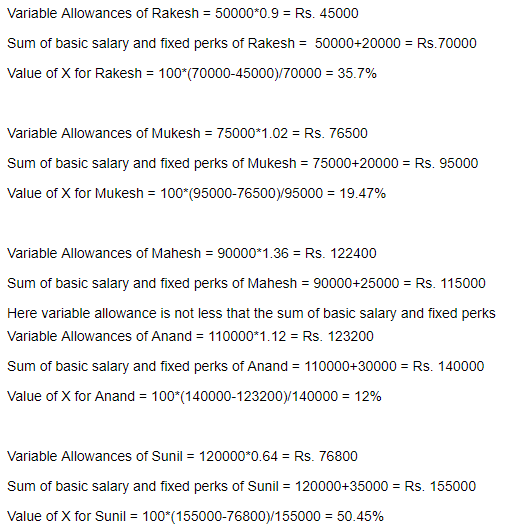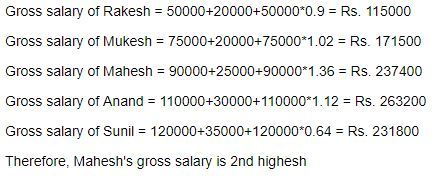# Quantitative Aptitude Practice Questions for IBPS PO Mains – Set 5

Quantitative Aptitude Practice Questions for IBPS PO Mains – Set 5
Directions (1 – 5): Study the following graph and answer the questions below:
Percentage number of students playing three different games in five different schools.

1. If the total number of students in college B are 4600 and the number of students in college C are 5 1/23% more than the number of students in college B then find the ratio of the students who play cricket from college B to the number of students who play football from college C ?
a) 4125 : 8889
b) 4025 : 8758
c) 8758 : 4025
d) 8889 : 4125
e) None of these
2. Number of students who play Cricket from college B are what % less than the number of students who play Tennis and Footbal from the same college.
a) 59 2/3%
b) 61 1/9%
c) 63 4/9%
d) 62 2/3%
e) None of these
3. Number of males who likes football from college D is same as number of females who likes Football from same college then find number of females who play football are what % of number of students who play Tennis from the same college ?
a) 21%
b) 23%
c) 20%
d) 14%
e) None of these
4. Find the average of the number of students who likes football and cricket from school C together if total number of students from college C are 81 11/69% of 6900.
a) 2240
b) 2245
c) 2255
d) 2250
e) 2247
5. Average number of student from college A and college E are 1240 and the ratio of the number of students from college A and college E are 3 : 2. Then number of students who likes football from college A are approximately what percent of the number of students who likes Tennis from college E ?
a) 246.12%
b) 243.63%
c) 246.43%
d) 256.20%
e) None of these
Directions (6 – 10): A company hired 5 new employees and offered them different salary.
The gross salary of an employee consists of basic salary, fixed perks and variable allowances. Every employee gets 5 types of variable allowances as different percentages of Basic Salary
The Table-1 (Figures in Rs.) gives Basic Salary and fixed perks of each employee and Bar-graph gives the breakup of variable allowances of each employee in percentage of basic salary
 Name Basic Salary Fixed Perks Rakesh 50000 20000 Mukesh 75000 20000 Mahesh 90000 25000 Anand 110000 30000 Sunil 120000 35000
6. If total variable allowances is X% less than the sum of basic salary and fixed perks, then for which employee the value of X is maximum?
a) Rakesh
b) Mukesh
c) Mahesh
d) Anand
e) Sunil
7. Which employee gets the 2nd highest gross salary?
a) Rakesh
b) Mukesh
c) Mahesh
d) Anand
e) Sunil
8. Which employee receives maximum variable allowance?
a) Rakesh
b) Mukesh
c) Mahesh
d) Anand
e) Sunil
9. The total expense by company on BDA of the five employees is approximately what percentage of total expenses on LTA of the five employees?
a) 75.4%
b) 132.6%
c) 85.5%
d) 112.8%
e) 97.2%
10. What is the ratio of total expense of company on basic salary and fixed perks to that of total expense of company on variable allowances over the five employees?
a) 125:126
b) 193:250
c) 250:193
d) 252:193
e) 193:252
Directions (11 – 15): Read the following graph carefully and answer the questions that follow.
75 million bottles of colas were consumed in 1984 and 115 million in 1998.
11. If Coke’s shares were to increase by 10% in 1999 with total sales remaining the same, what would be the percentage reduction in the total shares of other colas?
a) 1.7%
b) 4.2%
c) 6.1%
d) 3.3%
e) 2.9%
12. If Coke’s sales for 1998 had been 30 million bottles, then with the distribution shown in the chart remaining the same, the total sales in 1998 would be greater/lower than the actual value by what percentage?
a) +40%
b) +95%
c) +86%
d) +92%
e) None of these
13. What is the Approximate central angle of the Coke sector in 1984?
a) 80 degrees
b) 75 degrees
c) 79 degrees
d) 78 degrees
e) None of these
14. In 1999, if Parle’s market share was to become 30%, in a market of 125 million bottles, what would be Approximate Parle’s percentage gain over 1998 sales?
a) 27%
b) 30%
c) 33%
d) 25%
e) None of these
15. The increase in the consumption of Parle drinks was (in million):
a) 14.8
b) 16.9
c) 15.25
d) 17.8
e) None of these

Solutions:
1. B) No. of students in college C = 105 1/23% of 4600 = 4832
Required ratio = (28/100 × 4600) : (58/100 × 4832) = 1288 : 2802.52 = 4025 : 8758
2. B) Required % = (72 – 28)/72 × 100 = 61 1/9%
3. E) No. of females who play football from college D = 24/2% = 12%
Required % = 12/62 × 100 = 19 11/31%
4. A) Total no.of students from college = 81 11/69% of 6900 = 5600
Required average = 1/2 [(58 + 22)/100 × 5600] = 1/2 × 4480 = 2240
5. C) No. of students from college A = 3/5 × (1240 × 2) = 1488
No. of students from college E = 2/5 × (1240 × 2) = 992
Required % = [(46/100 × 1488)/(28/100 × 992)] × 100 = 246.43%
6. E)7. C)8. D)9. B)10. C)11. A) Total sales of coke in 1998 = 14 % of 115 million
= 14/100 × 115 = 16.1 million
Total sales of remaining cola in 1998 = 115-16.1 = 98.9 million
Now, Cokes’ share increase by 10 % resulting in sales = 16.1 + 10% of 16.1 = 17.71 million
Sales of remaining colas in 1999 = 115 – 17.71 million = 97.29 million
Difference in sales of remaining colas = 98.9 - 97.29 million
12. C) Percentage sales of coke in 1998 = 14%
Actual sales of coke in 1998 = 14/100 × 115 = 16.1 million
Given sale = 30 million
Difference = (30 – 16.1) = 13.9 million
Percentage of change = 13.9/16.1 × 100% = 86.33%
13. C) Share of coke in 1984 = 22 %
Angle subtended by coke sector in 1984 = 22/100 × 360 = 79.2 ≈ 79°
14. B) Scenario 1 – 1998
Share of Parle in total sales = 25%
Number of bottles of Parle sold = 25/100 × 115 million = 28.75 million
Scenario 2 – 1999
Share off Parle in total sales = 30% of 125 milion
Number of bottles of Parle sold = 30/100 × 125 million = 37.5 million
Increase = 37.5 – 28.75 million = 8.75 million
Percentage increase = 8.75/28.75 × 100 = 30.4 % ~ 30 %
15. C) Scenario 1 – 1984
Share of parle in total sales 18%
Number of bottles of parle sold = (18/100) × 75 million = 13.5 million
Scenario 2 – 1998
Share off parle in total sales = 25%
Number of bottles of parle sold = (25/100) × 115 million = 28.75 million
Increase = 28.75 – 13.5 million = 15.25 million